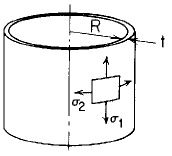Related Resources: pressure-vessel

### Cylinder Stress and Deflection Rotation Equation and Calculator

Cylinder Stress and Deflection Uniform rotation, ω rad/sec about central axis Equation and Calculator.

Per. Roarks Formulas for Stress and Strain for membrane stresses and deformations in thin-walled pressure vessels.

 Cylindrical( R / t ) > 10 Uniform rotation, ω rad/sec about central axisMeridional Stress at top
σ1 = 0

Circumferential or Hoop StressChange in height dimension yRotation of a meridian from its unloaded position
ψ = 0

Where used:
E = Modulus of Elasticity (lbs/in2)